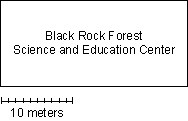### Black Rock Forest Electrical Energy Consumption versus Solar Energy Incidence

#### Worksheet #3. Compare Solar Incidence and Electrical Energy & Calculate Solar Panel Efficiency. ....You do the Math.

This worksheet will guide you in making a quantitative comparison between the electrical energy consumed by the Black Rock Forest Science & Education Center (SEC) and the solar energy received by the Forest.

Directions: Use the pre-selected data tables for February 2001 together with the Conversion Factors and the Fact Sheet. Use Excel or your calculator to find answers.

Step 1. Use the data to find the total amount of electrical energy used by the SEC for February 2001.

__________ kWH

Step 2: Based on the data, how many kWH of solar energy were incident on each square meter of Black Rock Forest in February 2001? Explain how you got your answer.

__________ kWH/m2

Step 3. Use the data to estimate the total amount of solar energy falling on the SEC during Feb 2001:

____________ _______ (units)

What are the units of this answer?

Step 4: Based on the data, is the amount of solar energy arriving on the SEC more, less or about the same as the amount of electrical energy used inside the building?

__________________

Step 5: In February 2001, how much land area would have been needed to collect sunlight with the same amount of energy as the amount of electrical energy used by the SEC building? Show your calculations.

____________ _______ (units)

What are the units of this answer?

Step 6. Imagine this area as circular in form, and find the radius of the circle with this area.

A = P r 2

radius = ___________ meters

Step 7. On the scaled diagram of the footprint of the SEC, sketch a circle to show the area on which the incident solar energy equals the amount of energy consumed as electricity inside the building.Query: What are your observations? What can you infer from your calculations?

Step 8: Repeat steps 1-6 for the data for the month of June 2001.

Step 9: Finally, we will answer the question, "How much of the electrical power of the building could be supplied by solar panels, if the average solar panel has a 10% efficiency?".

#### Guided Calculation for Solar Panel Efficiency

 1. SI for February (from step 3) _______________ 2. Multiply by footprint of the building x _______________ = _______________ 3. Multiply by solar efficiency % x .10 = _______________

Could solar panels provide the energy needed by the building?

What percent of the energy needed by the building in February could be provided by solar panels on the roof?

Repeat calculation for the month of June. Answer the same questions.